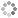# PROWAREtecharticles » current » assembly » x86 » calling-conventions-cdecl-versus-stdcall

## x86 Assembly: Calling Conventions - cdecl versus stdcall

Notes and examples of __cdecl (c-declaration) and __stdcall (standard-call) calling conventions.

Use Visual C++ Express Edition 2005 for MASM development.

`__cdecl` is the default calling convention for C/C++ programs because it allows for variable argument functions, such as `printf()`. `__cdecl` creates larger executables because the calling code must clean up the stack whereas with the `__stdcall` (standard-call) calling convention, the stack is cleaned up inside the routine being called.

A `__cdecl` procedure:

``````TITLE 'extern "C" int __cdecl subtract_cdecl(int a, int b);'
.386P
.model FLAT
PUBLIC	_subtract_cdecl
_TEXT	SEGMENT
_subtract_cdecl PROC NEAR

mov	eax, DWORD PTR [esp+4]	; move a to eax
sub	eax, DWORD PTR [esp+8]	; subtract b from eax

ret	0
_subtract_cdecl ENDP
_TEXT	ENDS
END
``````

A `__stdcall` procedure:

``````TITLE 'extern "C" int __stdcall subtract_stdcall(int a, int b);'
.386P
.model FLAT
PUBLIC	_subtract_stdcall@8
_TEXT	SEGMENT
_subtract_stdcall@8 PROC NEAR

mov	eax, DWORD PTR [esp+4]	; move a to eax
sub	eax, DWORD PTR [esp+8]	; subtract b from eax

ret	8
_subtract_stdcall@8 ENDP
_TEXT	ENDS
END
``````

An example of calling both `__cdecl` and `__stdcall` conventions:

``````TITLE 'main procedure'
.386P
.model FLAT
PUBLIC	_main
EXTRN	_subtract_cdecl:NEAR
EXTRN	_subtract_stdcall@8:NEAR
_TEXT	SEGMENT
_main	PROC NEAR

; subtract_cdecl(2, 3)

push	3
push	2
call	_subtract_cdecl
add	esp, 8              ; MUST CLEAN UP THE STACK AFTER EVERY __cdecl CALLING

; subtract_stdcall(20, 30)

push	30
push	20
call	_subtract_stdcall@8

; return 0;

xor	eax, eax

ret	0
_main	ENDP
_TEXT	ENDS
END
``````This site uses cookies. Cookies are simple text files stored on the user's computer. They are used for adding features and security to this site. Read the privacy policy.